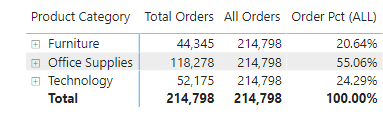Search

# Calculating % with DAXSomeone utterly new to Power BI and especially those who have been using Excel, understanding DAX can be an overwhelming experience. At least, it was for me. It took me some time to grasp the concepts, filter and row contexts, and the CALCULATE function.One of the standard requirements in a BI report is calculating percentages.

Let us consider simple visual showing orders by Product Category and percentage contribution:Achieving this is a reasonably easy task in Excel, and when using simple implicit measures in Power BISo how could we achieve the same using measures (DAX)? Well, this depends on how we want to see our results.

## Objective 1: Calculating the percentage of the total (ALL)

For calculating the percentage of the total, here is the formula: Total Orders by each Product / Total Orders

`Total Orders = SUM(Orders[Order Quantity])`

50% of the objective accomplished.

Now for the most uncomplicated part:

There are two evaluation contexts in Power BI/Tabula model: Filter Context and Row Context.

Evaluation of each DAX expressions happens inside a context.

A more natural way of understanding a filter context: In this example, when we add Product Category to the visual, it filters the Order table by each product category, and then calculate the sum of Order Quantity. A separate blog covers these contexts in detail.

The denominator of the ratio needs to ignore the existing filter context for calculating the overall total (or simply put, we need ALL the Product Categories). So, our formula becomes:

```All Orders =
CALCULATE(
[Total Orders],
ALL(Orders)
)```

In simple English, we are asking DAX to:

• Remove all the external filter contexts applied by the visual (Product Category)

• Calculate the sum of the Order Quantity of the visible columns.So, we have the numerator and denominator. The following measure calculates the required percentage:

```Order Pct (ALL) =

//Calculating the numerator
VAR _Orders =
[Total Orders] -- This measure calculates orders withing the filter context

//Calculating the denominator by removing any filter context
VAR _AllOrders =
CALCULATE(
[Total Orders],
ALL(Orders) –- Considers the entire Order table
)

//Calculating the ratio
VAR _OrderPct =
DIVIDE(_Orders, _AllOrders)

RETURN

_OrderPct```

And calling the measure in the visual:## Objective 2: Consider the filters applied to the visual (ALLSELECTED)

Let’s take one step further. We included a slicer in our report, and we wish to update the denominator according to the selection. We have learned in the previous segment; ALL removes all the external filters applied on the table during the calculation. The same is evident in the example below:The value of total orders remained unchanged even when additional filters were applied using a slicer.

```AllSelected Orders =
CALCULATE(
[Total Orders],
ALLSELECTED(Orders[Product Category]) -- Considers the filters applied by selecting any visual
)```In simple English, we are asking DAX to:

• Remove all the external filter contexts applied by the visual (Product Category)

• Filter the Product Category column in the Orders table on the selected values (Furniture & Office Supplies in this case)

• Calculate the sum of the Order Quantity of the visible columns.

Next step is to calculate the percentage:

```Order Pct (ALLSELECTED) =
DIVIDE([Total Orders], [AllSelected Orders])```## Objective 3: How to calculate the percentage of the parent total? (ALLEXCEPT)

When dealing with hierarchical data, the requirement is to calculate % of the parent total:In the example above, there are two levels of filter contexts applied:

• Level 1: Product Category

• Level 2: Product Sub-Category

The objective is to keep filters at Level 1 and not at Level 2. ALLEXCEPT comes to the rescue:

```AllExcept Orders =
CALCULATE(
[Total Orders],
ALLEXCEPT(Orders, Orders[Product Category])
)```

In simple English, we are asking DAX to:

• Remove all the external filter contexts applied by the visual (Product Category & Sub-Category)

• Filter the Product Category column in the Orders table on the Product Category only

• Calculate the sum of the Order Quantity of the visible columns.

And then we use the DIVIDE function to calculate the percent contribution:

```Order Pct (ALLEXCEPT) =
DIVIDE([Total Orders], [AllExcept Orders])```

In conclusion, if we know how we want to filter our data table and take the help of appropriate DAX, calculating the percentage of the total is not that complicated.

Simple when you know how.

@imVivRan